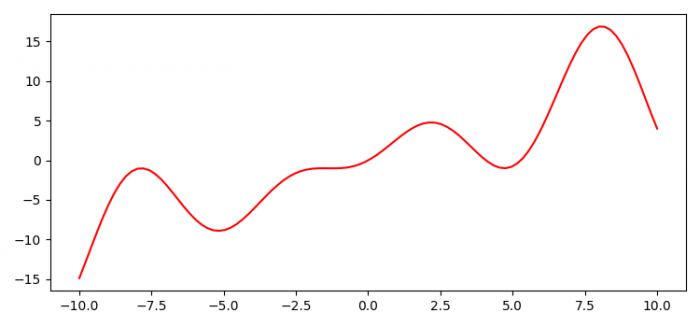# How to plot a function defined with def in Python? (Matplotlib)

To plot a function defined with def in Python, we can take the following steps −

• Set the figure size and adjust the padding between and around the subplots.
• Create a user-defined function using, def, i.e., f(x).
• Create x data points using numpy.
• Plot x and f(x) using plot() method.
• To display the figure, use show() method.

## Example

import numpy as np
from matplotlib import pyplot as plt

plt.rcParams["figure.figsize"] = [7.50, 3.50]
plt.rcParams["figure.autolayout"] = True

def f(x):
return np.sin(x) + x + x * np.sin(x)

x = np.linspace(-10, 10, 100)

plt.plot(x, f(x), color='red')

plt.show()

## Output